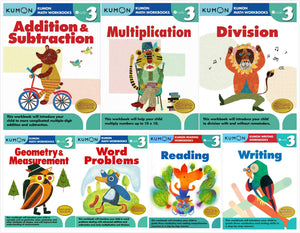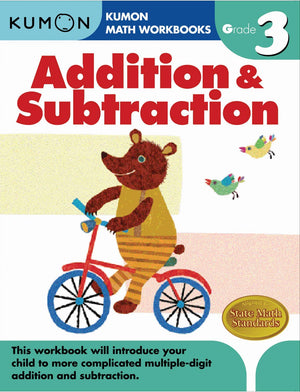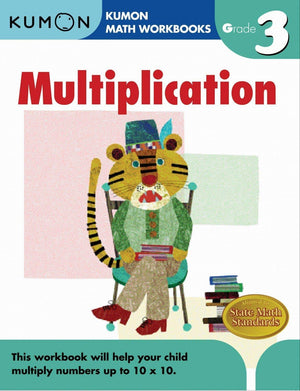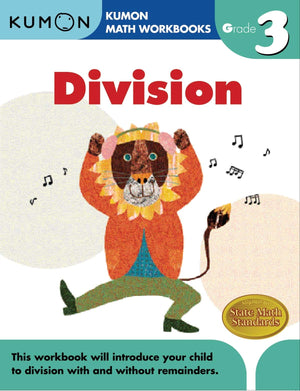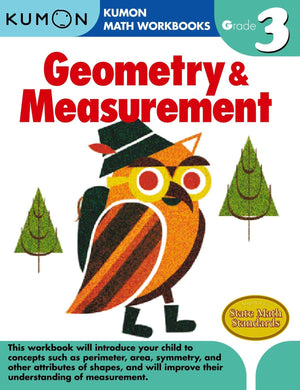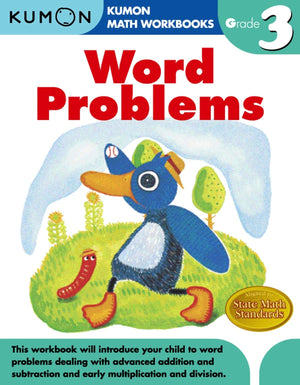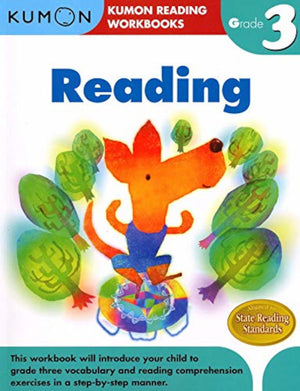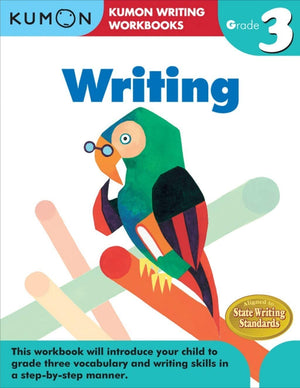# Kumon Grade 3 Math Workbooks and More (Set of 7)

Regular price \$59.95
Unit price  per

Kumon Grade 3 Math Workbooks and More (Set of 7): Addition & Subtraction, Multiplication, Division, Geometry & Measurement, Word Problems, Reading, and Writing

This Kumon set includes the following 7 grade 3 books: Addition & Subtraction, Multiplication, Division, Geometry & Measurement, Word Problems, Reading, and Writing. Helps third graders and kids in homeschool with their basic subjects and curriculum! Study mathematics and practice handwriting on the simple worksheets within the book! Addition & Subtraction: Workbook review helps with comprehension. Four-digit vertical form addition. Four-digit vertical form subtraction. Regrouping (borrowing). Division: Topics Covered in this Book: Review of multiplication. Inverse relationship of multiplication & division. Two-digit by one-digit vertical form division. Division with remainders -Early three- & four-digit by one-digit division. Identity property of division. Zero property of division. Patterns & sequencing. Geometry & Measurement: Ordinal numbers. Compose and decompose two-dimensional shapes. Group two-dimensional objects and shapes by like properties. Length (inch, foot, yard, centimeter, meter). Weight (pound, gram, kilogram). Capacity (pint, quart, liter). Time (to five minutes). Temperature. Money (coins and bills, \$1, \$5, \$10, \$20). Find the area. Multiplication: Repeated addition. Skip counting. Multiplication facts to 10 x 10. Inverse relationship of multiplication & division. Commutative property of multiplication. Identity property of multiplication. Zero property of multiplication. Word Problems: helps your child build a strong foundation in word problems with multiplication and division. Then, by mastering graphs and tables, your child will advance to more difficult word problems with ease. Reading: Vocabulary Building. Prefixes & Suffixes. Compare & Contrast. Defining Words by Context. Who, When, Where, What, Why, & How. Chart the Passage. Sequencing. Making & Revising Predictions. Reading Storie. Writing: your child will write one and two paragraphs with ease. Declarative, interrogative, imperative, are introduced through our step-by-step progression.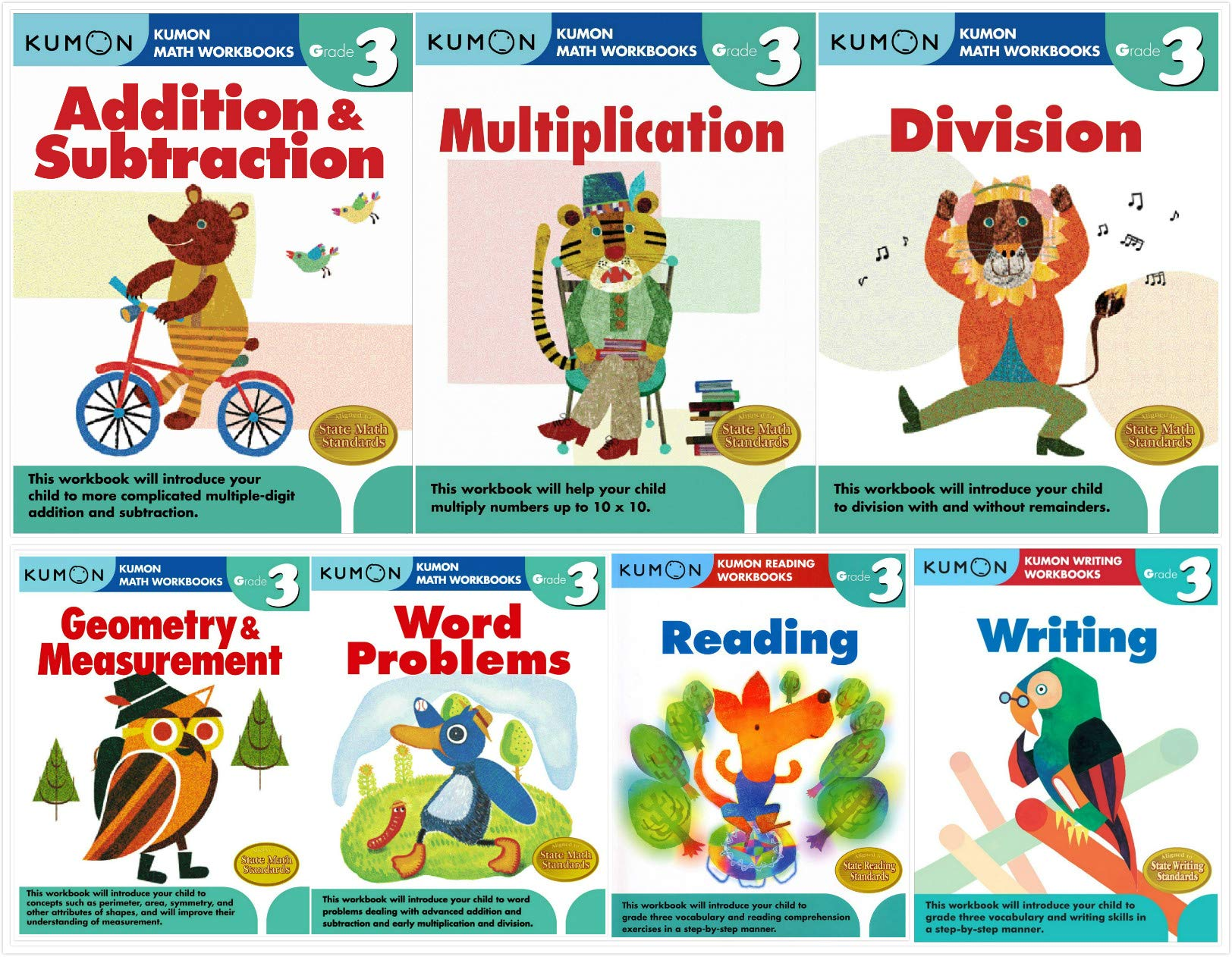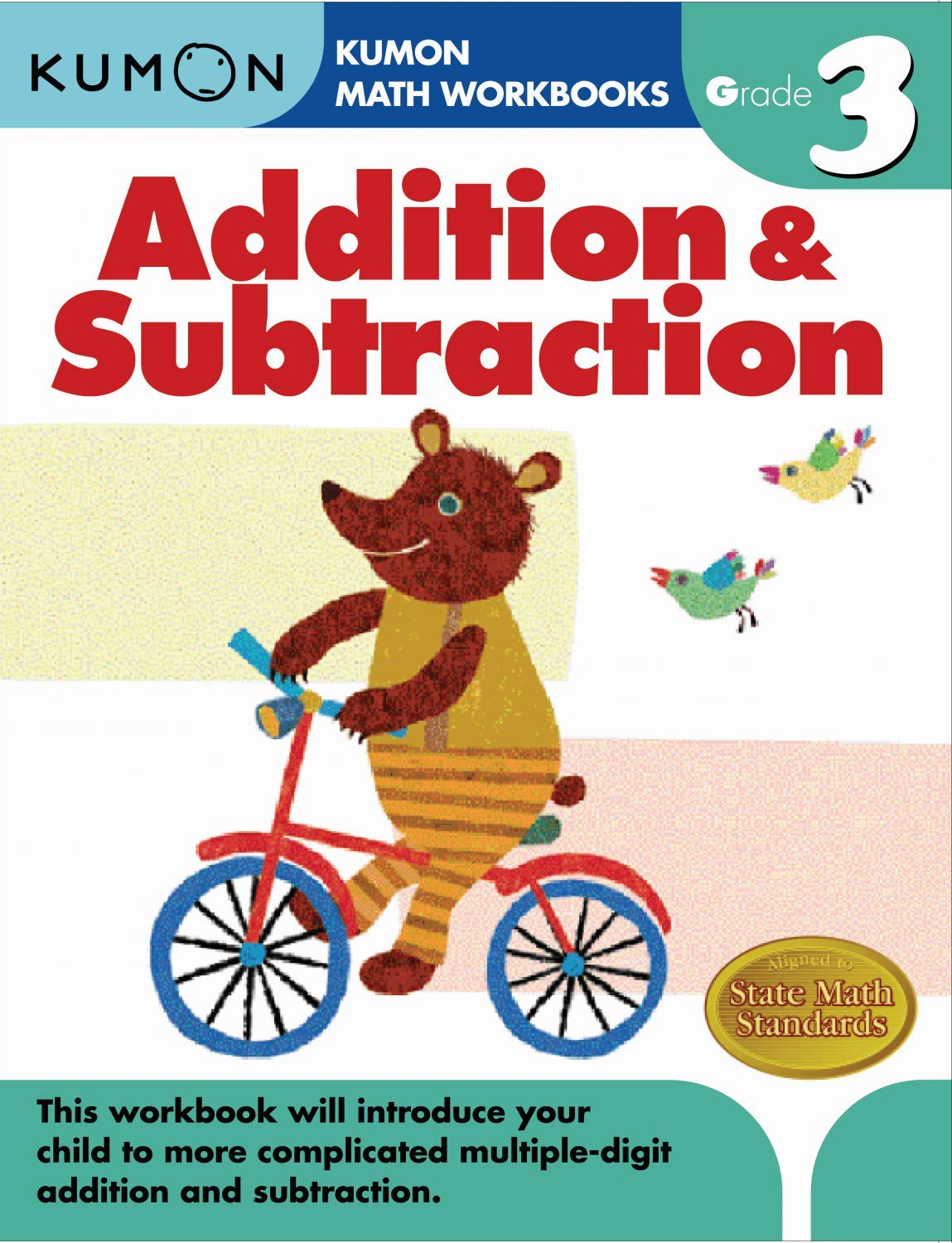Kumon